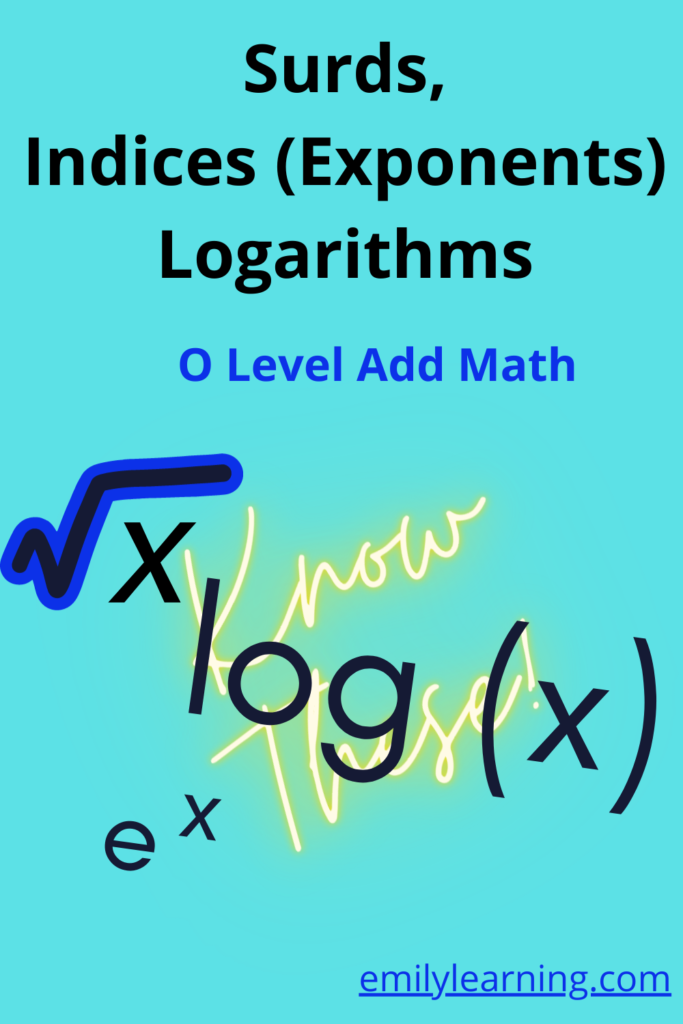# What you need to know for O Level for Surds, Indices and Logarithms (O Level Add Maths)Surds, indices, and logarithms are chapters that are tested in O Level Additional Mathematics.

You also need to apply what you have learnt in these chapter to other chapters like differentiation since there is a chapter on differentiation of exponential and lograithmic function.

Since this post is on what you need to know specifically on surds, indices and logarithms, I will skip the portion on differentiation. Check out our post on what you need to know for differentiation in O Level Additional Mathematics to find out more.

So here’s what you need to know for the topic on surds, indices and logarithms in O Level Additional Mathematics:

Surds:

• add, subtract, divided and multiply (4 operations) surds
• rationalize denominators involving surds
• solving equations involving surds

Exponents:

• graphs of exponents
• how to simplify expressions involving exponents (your elementary mathematics knowledge comes in)
• simplify expressions involving exponents
• solving equations involving exponents (sometimes you’ll need to convert the exponent expression to a logarithmic expression)

Logarithm:graphs of logarithmlaws of logarithmchange of base of logarithmsimplify expressions involving logarithm solving equations involving logarithm

## Want to learn O Level Additional Math on-demand?

Check out our on-demand O Level Additional Math course here.

error: Content is protected !!